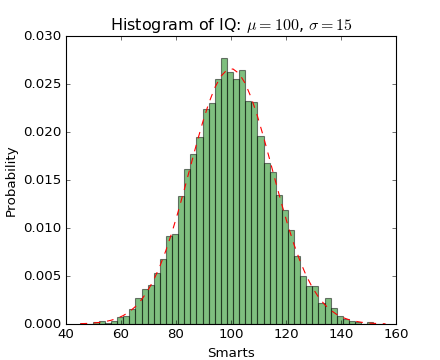You are reading an old version of the documentation (v1.5.3). For the latest version see https://matplotlib.org/stable/

## Matplotlib 2.0.0rc2 is available

Install the release candidate now!Travis-CI:# statistics example code: histogram_demo_features.py¶"""
Demo of the histogram (hist) function with a few features.

In addition to the basic histogram, this demo shows a few optional features:

* Setting the number of data bins
* The normed flag, which normalizes bin heights so that the integral of
the histogram is 1. The resulting histogram is a probability density.
* Setting the face color of the bars
* Setting the opacity (alpha value).

"""
import numpy as np
import matplotlib.mlab as mlab
import matplotlib.pyplot as plt

# example data
mu = 100  # mean of distribution
sigma = 15  # standard deviation of distribution
x = mu + sigma * np.random.randn(10000)

num_bins = 50
# the histogram of the data
n, bins, patches = plt.hist(x, num_bins, normed=1, facecolor='green', alpha=0.5)
# add a 'best fit' line
y = mlab.normpdf(bins, mu, sigma)
plt.plot(bins, y, 'r--')
plt.xlabel('Smarts')
plt.ylabel('Probability')
plt.title(r'Histogram of IQ: $\mu=100$, $\sigma=15$')

# Tweak spacing to prevent clipping of ylabel